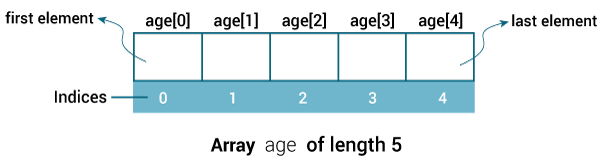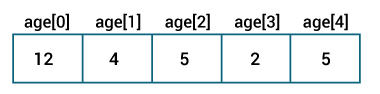# An array is a container that holds data (values) of a single type.

For example, you can create an array that can hold 100 int values.

An array is a basic structure in Java that allows you to easily store and access large amounts of values.

## How to define an array?

Here is how to define an array in Java:

dataType [] arrayName;

• dataType can be a raw data type such as: int, char, Double, byte, etc. or an object
• arrayName is the identifier.

Let us repeat the above example.

double [] data;

Here data is an array that can hold values ​​of type Double.

But how many elements can the data array hold?

That was a good question! We have not defined it yet. The next step is to allocate memory for the array elements.

data = new Double ;

The length of the data array is 10. This means that it can hold 10 elements (in this case 10 double values).

Note that once the array length is defined, it cannot be changed during the program.

Let’s take another example:

int [] age;

age = new int ;

Here, the age array can hold 5 values ​​of type int.

In one command, the memory of an array can be declared and allocated. You can replace the above two sentences with a single sentence.

int [] age = new int ;

## Array Index in Java

You can access elements of an array using indexes. Consider the previous example.

int [] age = new int ;The first element of the array is [age , the second element [age  and …

If the array length is n, the last element will be [arrayName] n-1. Since the length of the age array is 5, the last element of the array in the example above is age.

The default initial value of the elements of an array is 0 for all numeric types and false for boolean. Consider the following example:

1. class ArrayExample {
2. public static void main (String [] args) {
3. int [] age = new int ;
4. System.out.println (age );
5. System.out.println (age );
6. System.out.println (age );
7. System.out.println (age );
8. System.out.println (age );
9. }
10. }

Output

۰

۰

۰

۰

۰

There is a better way to access the elements of an array using a loop structure (usually a for loop).

1. class ArrayExample {
2. public static void main (String [] args) {
3. int [] age = new int ;
4. for (int i = 0; i <5; ++ i) {
5. System.out.println (age [i]);
6. }
7. }
8. }

## How to initialize arrays in Java?

In Java, you can set arrays when defining, or you can later value or modify the values ​​to suit your needs.

Initialize the array when defining

Here’s how to set an array when defining.

int [] age = {12, 4, 5, 2, 5};

This expression creates an array and values ​​it when defining it.

The length of the array is determined by the number of values ​​separated by commas. In this example, the length of the array is 5.Let’s write a simple program to print the elements of this array.

1. class ArrayExample {
2. public static void main (String [] args) {
3. int [] age = {12, 4, 5, 2, 5};
4. for (int i = 0; i <5; ++ i) {
5. System.out.println (“Element at index” + i + ”:” + age [i]);
6. }
7. }
8. }

The output of the above code is equal to:

Element at index 0:12

Element at index 1: 4

Element at index 2: 5

Element at index 3: 2

Element at index 4: 5

## How to access array elements?

Using its numeric list, you can easily access and modify array elements. Let’s give an example.

1. class ArrayExample {
2. public static void main (String [] args) {
3. int [] age = new int ;
4. // insert 14 to third element
5. age  = 14;
6. // insert 34 to first element
7. age  = 34;
8. for (int i = 0; i <5; ++ i) {
9. System.out.println (“Element at index” + i + ”:” + age [i]);
10. }
11. }
12. }

Output

Element at index 0:34

Element at index 1: 0

Element at index 2:14

Element at index 3: 0

Element at index 4: 0

#### Example: Arrays in Java

The following program calculates the sum and average of the values ​​stored in an int array.

1. class SumAverage {
2. public static void main (String [] args) {
3. int [] numbers = {2, -9, 0, 5, 12, -25, 22, 9, 8, 12};
4. int sum = 0;
5. Double average;
6. for (int number: numbers) {
7. sum + = number;
8. }
9. int arrayLength = numbers.length;
10. // Change sum and arrayLength to double as average is in double
11. average = ((double) sum / (double) arrayLength);
12. System.out.println (“Sum =” + sum);
13. System.out.println (“Average =” + average);
14. }
15. }

#### Output

Sum = 36

Average = 3.6

• The for..each loop is used to access each element of the array.
• To calculate the mean, the integers sum and arrayLengh are doubled because the mean is double. This is called data type conversion.
• The lenght property is used to get the array length. Here, numbers.lenght returns the length of the array of numbers.

## Multidimensional arrays

The arrays we have mentioned so far are called one-dimensional arrays. In Java, you can define an array of arrays known as multidimensional arrays. Here is an example to define and initialize a multidimensional array.

Double [] [] matrix = {{1.2, 4.3, 4.0},

{4 ٫ 1, -1 ٫ 1}

};

Here, the matrix is ​​a 2D array.